# Bijective function facts for kids

Kids Encyclopedia Facts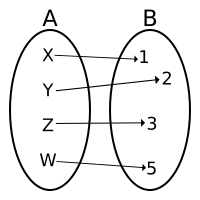Bijection. There is exactly one arrow to every element in the codomain B (from an element of the domain A).

In mathematics, a bijective function or bijection is a function f : AB that is both an injection and a surjection. This is equivalent to the following statement: for every element b in the codomain B, there is exactly one element a in the domain A such that f(a)=b. Another name for bijection is 1-1 correspondence (read "one-to-one correspondence).

The term bijection and the related terms surjection and injection were introduced by Nicholas Bourbaki. In the 1930s, he and a group of other mathematicians published a series of books on modern advanced mathematics.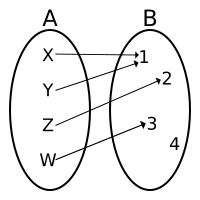Not a bijection. (It is not a surjection. It is not an injection.)

## Basic properties

Formally: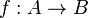$f:A \rightarrow B$ is a bijective function if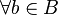$\forall b \in B$, there is a unique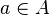$a \in A$ such that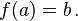$f(a)=b \,.$

where the element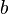$b$ is called the image of the element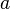$a$, and the element$a$ the pre-image of the element$b$.

The formal definition can also be interpreted in two ways:

• Every element of the codomain B is the image of exactly one element in the domain A.
• Every element of the codomain B has exactly one pre-image in the domain A.

Note: Surjection means minimum one pre-image. Injection means maximum one pre-image. So bijection means exactly one pre-image.

## Cardinality

Cardinality is the number of elements in a set. The cardinality of A={X,Y,Z,W} is 4. This can be written as #A=4.

By definition, two sets A and B have the same cardinality if there is a bijection between the sets. So #A=#B means there is a bijection from A to B.

## Bijections and inverse functions

Bijections and inverse functions are related to each other, in that a bijection is invertible, can be turned into its inverse function by reversing the arrows.

Formally: Let f : AB be a bijection. The inverse function g : BA is defined by if f(a)=b, then g(b)=a. (See also Inverse function.)

• The inverse function of the inverse function is the original function.
• A function has an inverse function if and only if it is a bijection.

Note: The notation for the inverse function of f is confusing. Namely,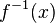$f^{-1}(x)$  denotes the inverse function of the function f, but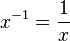$x^{-1}=\frac{1}{x}$ denotes the reciprocal value of the number x.

## Examples

### Elementary functions

Let f(x):ℝ→ℝ be a real-valued function y=f(x) of a real-valued argument x. (This means both the input and output are numbers.)

• Graphic meaning: The function f is a bijection if every horizontal line intersects the graph of f in exactly one point.
• Algebraic meaning: The function f is a bijection if for every real number yo we can find at least one real number xo such that yo=f(xo) and if f(xo)=f(x1) means xo=x1 .

Proving that a function is a bijection means proving that it is both a surjection and an injection. So formal proofs are rarely easy. Below we discuss and do not prove. (See surjection and injection.)

Example: The linear function of a slanted line is a bijection. That is, y=ax+b where a≠0 is a bijection.

Discussion: Every horizontal line intersects a slanted line in exactly one point (see surjection and injection for proofs). Image 1.

Example: The polynomial function of third degree: f(x)=x3 is a bijection. Image 2 and image 5 thin yellow curve. Its inverse is the cube root function f(x)= ∛x and it is also a bijection f(x):ℝ→ℝ. Image 5: thick green curve.

Example: The quadratic function f(x) = x2 is not a bijection (from ℝ→ℝ). Image 3. It is not a surjection. It is not an injection. However, we can restrict both its domain and codomain to the set of non-negative numbers (0,+∞) to get an (invertible) bijection (see examples below).

Note: This last example shows this. To determine whether a function is a bijection we need to know three things:

• the domain
• the function machine
• the codomain

Example: Suppose our function machine is f(x)=x².

• This machine and domain=ℝ and codomain=ℝ is not a surjection and not an injection. However,
• this same machine and domain=[0,+∞) and codomain=[0,+∞) is both a surjection and an injection and thus a bijection.

### Bijections and their inverses

Let f(x):AB where A and B are subsets of ℝ.

• Suppose f is not a bijection. For any x where the derivative of f exists and is not zero, there is a neighborhood of x where we can restrict the domain and codomain of f to be a bisection.
• The graphs of inverse functions are symmetric with respect to the line y=x. (See also Inverse function.)

Example: The quadratic function defined on the restricted domain and codomain [0,+∞)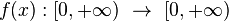$f(x):[0,+\infty) \,\, \rightarrow \,\, [0,+\infty)$  defined by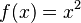$f(x) = x^2$

is a bijection. Image 6: thin yellow curve.

Example: The square root function defined on the restricted domain and codomain [0,+∞)$f(x):[0,+\infty) \,\, \rightarrow \,\, [0,+\infty)$  defined by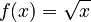$f(x) = \sqrt{x}$

is the bijection defined as the inverse function of the quadratic function: x2. Image 6: thick green curve.

Example: The exponential function defined on the domain ℝ and the restricted codomain (0,+∞)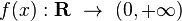$f(x):\mathbf{R} \,\, \rightarrow \,\, (0,+\infty)$  defined by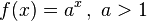$f(x) = a^x \, ,\,\, a>1$

is a bijection. Image 4: thin yellow curve (a=10).

Example: The logarithmic function base a defined on the restricted domain (0,+∞) and the codomain ℝ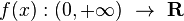$f(x):(0,+\infty) \,\, \rightarrow \,\, \mathbf{R}$  defined by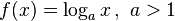$f(x) = \log_a x \, ,\,\, a>1$

is the bijection defined as the inverse function of the exponential function: ax. Image 4: thick green curve (a=10).

 Bijection: every vertical line (in the domain) and every horizontal line (in the codomain) intersects exactly one point of the graph.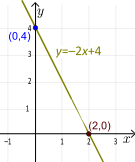1. Bijection. All slanted lines are bijections f(x):ℝ→ℝ.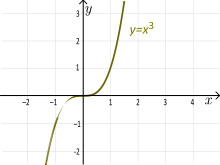2. Bijection. f(x):ℝ→ℝ. f(x)=x³.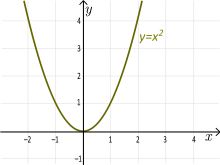3. Not a bijection. f(x):ℝ→ℝ. f(x)=x² is not a surjection. It is not an injection.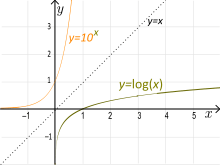4. Bijections. f(x):ℝ→ (0,+∞). f(x)=10x (thin yellow) and its inverse f(x):(0,+∞)→ℝ. f(x)=log10x (thick green).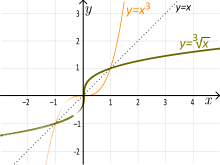5. Bijections. f(x):ℝ→ℝ. f(x)=x³ (thin yellow) and its inverse f(x)=∛x (thick green).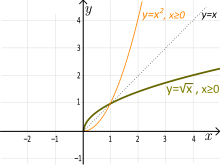6. Bijections. f(x):[0,+∞)→[0,+∞). f(x)=x² (thin yellow) and its inverse f(x)=√x (thick green).

## Related pages

interactive quiz interactiveBijective function Facts for Kids. Kiddle Encyclopedia.